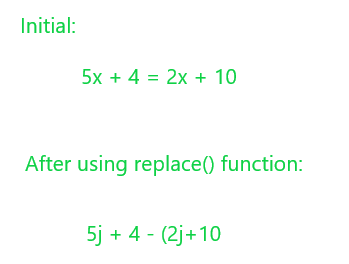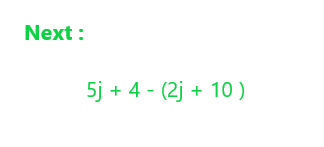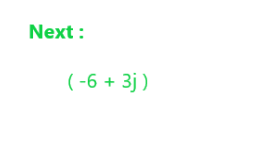# Solve Linear Equations using eval() in Python

• Difficulty Level : Medium
• Last Updated : 26 Mar, 2021

Linear equations using one variable of the form a + bx = c + dx can be solved in Python using eval() function. The input type will be a linear equation in the form of a string.

Syntax:

`eval(expression, globals=None, locals=None)`

Here, we will transform the equation into an expression of real and imaginary numbers such that eval can easily process it.

For example, 5x + 4 = 2x + 10 becomes 5j + 4 – (2j + 10) by shifting all the terms on the right side to the left. We are transforming this equation into a complex equation because eval() is unable to otherwise process the equations. Transforming it into a complex number helps in faster evaluation.

Step 1: We will use the replace() in python to replace “=” with “-(” and replace “x” with “j”.Step 2: The string is then added with “+)” to complete the expression.Step 3: Then { “j” : 1j} is done to change the equation into a format that can be easily evaluated by the eval() function. In this step all the constant terms are evaluated and the x terms or the imaginary terms as well.Step 4: Then the evaluated expression is simply broken down into the real and imaginary parts. If the imaginary part exists or the x is true and not zero the answer is printed else if the imaginary part is 0 and the real part is true there is no solution or else there are infinite solutions. Here,

`x = 2.000000`

is the final solution.

Example 1:

## Python3

 `def` `solve(equation):``   ` `    ``# replacing all the x terms with j ``    ``# the imaginary part``    ``s1 ``=` `equation.replace(``'x'``, ``'j'``)``     ` `    ``# shifting the equal sign to start ``    ``# an opening bracket``    ``s2 ``=` `s1.replace(``'='``, ``'-('``)``     ` `    ``# adding the closing bracket to form ``    ``# a complete expression``    ``s ``=` `s2``+``')'``     ` `    ``# mapping the literal j to the complex j``    ``z ``=` `eval``(s, {``'j'``: ``1j``})``    ``real, imag ``=` `z.real, ``-``z.imag``     ` `    ``# if the imaginary part is true return the``    ``# answer``    ``if` `imag:``        ``return` `"x = %f"` `%` `(real``/``imag)``    ``else``:``        ``if` `real:``            ``return` `"No solution"``        ``else``:``            ``return` `"Infinite solutions"`` ` ` ` `equation ``=` `"2+3x=5x-7"``print``(solve(equation))`

Output

`x = 4.500000`

Example 2:

## Python3

 `def` `solve(equation):``   ` `    ``# replacing all the x terms with j ``    ``# the imaginary part``    ``s1 ``=` `equation.replace(``'x'``, ``'j'``)``     ` `    ``# shifting the equal sign to start ``    ``# an opening bracket``    ``s2 ``=` `s1.replace(``'='``, ``'-('``)``     ` `    ``# adding the closing bracket to form ``    ``# a complete expression``    ``s ``=` `s2``+``')'``     ` `    ``# mapping the literal j to the complex j``    ``z ``=` `eval``(s, {``'j'``: ``1j``})``    ``real, imag ``=` `z.real, ``-``z.imag``     ` `    ``# if the imaginary part is true return the``    ``# answer``    ``if` `imag:``        ``return` `"x = %f"` `%` `(real``/``imag)``    ``else``:``        ``if` `real:``            ``return` `"No solution"``        ``else``:``            ``return` `"Infinite solutions"`` ` ` ` `equation ``=` `"x=x+10"``print``(solve(equation))`

Output

`No solution`

Example 3:

## Python3

 `def` `solve(equation):``   ` `    ``# replacing all the x terms with j``    ``# the imaginary part``    ``s1 ``=` `equation.replace(``'x'``, ``'j'``)``     ` `    ``# shifting the equal sign to start ``    ``# an opening bracket``    ``s2 ``=` `s1.replace(``'='``, ``'-('``)``     ` `    ``# adding the closing bracket to form ``    ``# a complete expression``    ``s ``=` `s2``+``')'``     ` `    ``# mapping the literal j to the complex j``    ``z ``=` `eval``(s, {``'j'``: ``1j``})``    ``real, imag ``=` `z.real, ``-``z.imag``     ` `    ``# if the imaginary part is true return the``    ``# answer``    ``if` `imag:``        ``return` `"x = %f"` `%` `(real``/``imag)``    ``else``:``        ``if` `real:``            ``return` `"No solution"``        ``else``:``            ``return` `"Infinite solutions"`` ` ` ` `equation ``=` `"2x=2x"``print``(solve(equation))`

Output

`Infinite solutions`

My Personal Notes arrow_drop_up